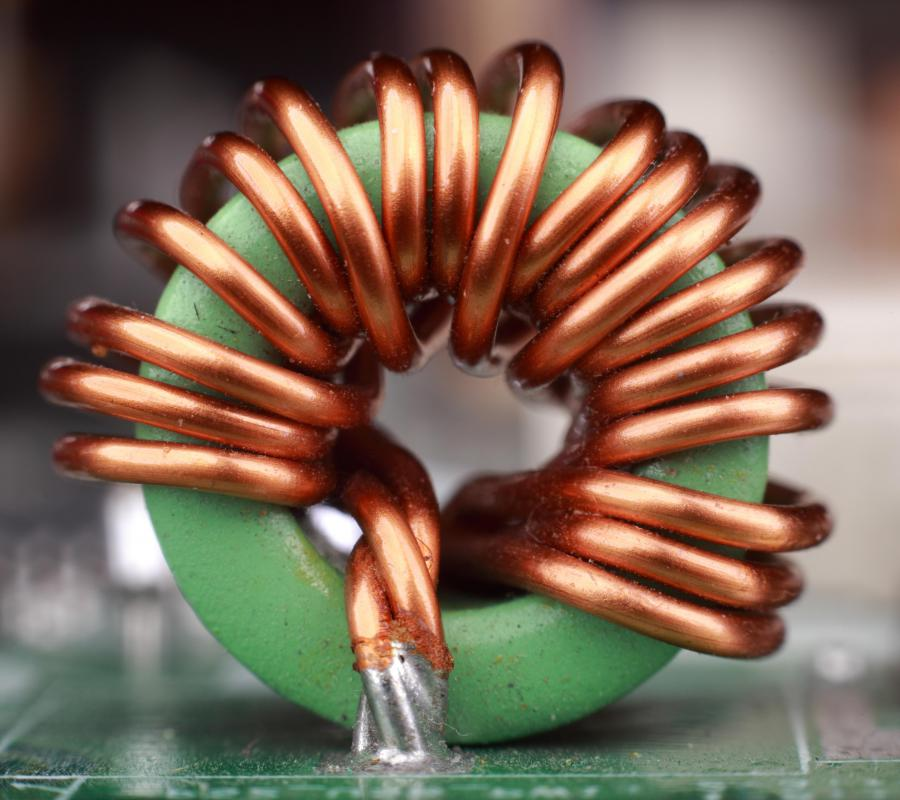# What is an Inductor Circuit? (with picture)

MJ Logan
MJ LoganCoils of copper wire are commonly used in electrical inductors.

Inductors are passive electronic components usually made from coils of wire. When an electric current passes through a coil of wire, or inductor, it induces a magnetic field around the coil, which stores energy. This energy storage ability is called inductance, and it is measured in henries. There are four main types of inductor circuits, and each behaves in a unique way that makes it useful in electronic circuits.

The magnetic field around an inductor stores energy. When the current is removed, the energy is reabsorbed by the inductor which produces a momentary current in the opposite direction of the original current. This current reacts with other components in the inductor circuit. Inductor circuit components include inductors (L), resistors (R) and capacitors (C). An RL inductor circuit, for example, has an inductor and a resistor in it.

Understand inductor circuits requires understanding that capacitors store energy in the form of an electrical charge placed on their plates. A capacitor's ability to store energy is called capacitance and is measured in farads. In an inductor circuit, a capacitor and an inductor store and discharge energy in opposition. As the magnetic field around an inductor builds, the capacitor charge is declining. The reverse also is true — as the capacitor charges, the inductor magnetic field declines.

A parallel resistor-inductor circuit is an isolator circuit for transistors used as amplifiers. At high frequencies, the transistor amplifier output begins to oscillate as the output capacitor stores and releases energy. A parallel resistor-inductor circuit connected across the amplifier output prevents the output from oscillating and distorting the signal or destroying components. It accomplishes this by absorbing energy as the capacitor discharges and discharging the energy as the capacitor charges, effectively keeping the transistor isolated from the shifting capacitor current.

The RL filter inductor circuit places an inductor and a resistor in series — the current flows through one, then the other. This circuit cam also be called a low-pass or high-pass filter, depending on how the output is taken from it. The high-pass filter application uses the inductor leads as the output, which allows high frequencies to pass but not low frequencies. Taking the output across the resistor uses the circuit as a low-pass filter, which passes low frequencies and blocks high frequencies.

Placing an inductor in parallel or in series with a capacitor creates a resonance circuit or tuned inductor circuit. The two components store and release energy in opposition — as one component is charging, the other is discharging. The LC inductor circuit is a selective filter, and the resonant frequency — the frequency at which both components charge and discharge equally — of the circuit selects the specific signal frequency that it allows to pass. This principle was the basis for early crystal radios that relied on a coil of wire and the capacitance of the antenna wire in the air to tune in different radio stations.

A simple RLC inductor circuit places the three components in series with each other. This circuit acts much like a series LC circuit in that it has a resonant frequency. Unlike the LC circuit, however, the series RLC circuit quickly loses the current oscillation between the capacitor and inductor because the resistor "resists" the flow of current. Other RLC inductor circuits place the components in different combinations of parallel and series circuits.

## You might also Like## Discuss this Article

Forgot password?
###### Register:
•Coils of copper wire are commonly used in electrical inductors.